# Unknown number 22

I am a two-digit number. My tens digits are 4. My one digit is three more than my tens. Who am I?

x =  47

### Step-by-step explanation: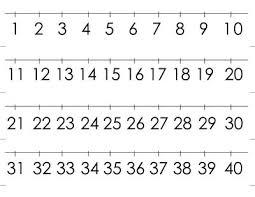We will be pleased if You send us any improvements to this math problem. Thank you!## Related math problems and questions:

• A five-digit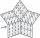A five-digit odd number has the sum of all digits (digits) five and contains two zeros. If we move each digit in the number one place to the left and move the first digit to the last place, we get a number 20,988 smaller. Find this unknown five-digit numb
• Two-digit numberDigit sum of thinking two-digit natural number is 11. When it exchanging a sequence of digits, given a number which is 27 less than the thinking number. Find out which number I think.
• Unknown number 16My number's tens is three times more then ones My number's ones is twice the number of thousands and my number's hundreds is half the number of of tens. I have two ones. which number am I?
• Four-digit numbers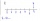Find four-digit numbers where all the digits are different. For numbers, the sum of the third and fourth digits is twice the sum of the first two digits, and the sum of the first and fourth digits is equal to the sum of the second and third digits. The di
• Five-digit numberAnna thinks of a five-digit number that is not divisible by three or four. If he increments each digit by one, it gets a five-digit number that is divisible by three. If he reduces each digit by one, he gets a five-digit number divisible by four. If it sw
• Unknown number 716% of the unknown number is by 21 less than unknown number itself. Determine the natural unknown number.
• Mystery numberA mystery number is between 800 and 920. No digits are the same. The difference of the numbers 5. What is the mystery number?
• Three-digit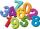How many three-digit natural numbers are greater than 321 if no digit in number repeated?
• Dd 2-digit numbersFind all odd 2-digit natural numbers compiled from digits 1; 3; 4; 6; 8 if the digits are not repeated.
• Unknown numberSamuel wrote unknown number. Then he had add 200000 to the number and the result multiply by three. When it calculated he was surprised, because the result would have received anyway, if write digit to the end of original number. Find unknown number.
• Three digits numberFrom the numbers 1, 2, 3, 4, 5 create three-digit numbers that digits not repeat and number is divisible by 2. How many numbers are there?
• No. 215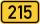From the number 215 we can create a four-digit number that among its numbers manually type any other digit. Thus, we created two four-digit numbers whose difference is 120. What two four-digit numbers that might be?
• How many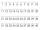How many double-digit numbers greater than 30 we can create from digits 0, 1, 2, 3, 4, 5? Numbers cannot be repeated in a two-digit number.
• Permutations with repetitionsHow many times the input of 1.2.2.3.3.3.4 can be permutated into 4 digits, 3 digits and 2 digits without repetition? Ex: 4 digits = 1223, 2213, 3122, 2313, 4321. . etc 3 digits = 122.212.213.432. . etc 2 digits = 12, 21, 31, 23 I have tried permutation fo
• Two-digit numberI am a two-digit number less than 20. When I divided by three, then you get the rest 1 and when you divide me by four you get also rest 1. What number am I?
• Result and remainderAfter dividing the unknown number by the number 23, the quotient 11 and the remainder 4 are formed. Find an unknown number.
• Divisible by fiveHow many different three-digit numbers divisible by five can we create from the digits 2, 4, 5? The digits can be repeated in the created number.